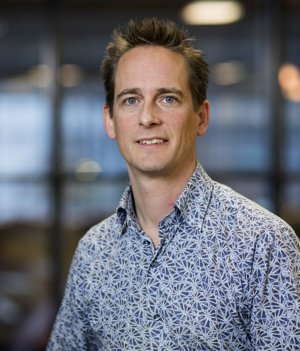# Dr.ir. Remco Duits (TU/e - CASA-GLDG)

Date: 12 January 2023

Time: 16.00-17.00 Hours

Room:  RA1501 & online

Speaker: Remco Duits,  (TU/e Department of Mathematics and Computer Science (CASA-GLDG)).## Title: “PDE-G-CNNs: PDE-based roto-translation equivariant convolutional neural networks and applications."

Abstract:
We consider PDE-based Group equivariant Convolutional Neural Networks (PDE-G-CNNs) that generalize Group equivariant Convolutional Neural Networks (G-CNNs). In PDE-G-CNNs a network layer is a set of PDE-solvers where geometrically meaningful PDE-coefficients become trainable weights. The underlying PDEs follow from scale space axioms and are morphological and linear scale space PDEs on the homogeneous space of positions and orientations. The network is solely implemented by 1) morphological convolutions with approximations to kernels solving nonlinear HJB-PDEs and by 2) linear convolutions solving linear PDEs.  The nonlinear morphological convolutions perform (soft) max-pooling over Riemannian balls. We prove that our analytic approximations of (sub)-Riemannian balls and kernels carry the correct symmetries and are accurate. PDE-G-CNNs do NOT include mystifying activations (like ReLU) and are geometrically interpretable: they efficiently train a sparse set of association fields from cortical modeling in neuro-geometry.

PDE-G-CNNs also have many practical benefits over G-CNNs: increase of classification performance along with a huge reduction in network complexity and training data. In this talk I focus on their benefits for geodesic tracking and segmentation of complex vasculature in medical images.